Ex 15.2 (Optional)

Chapter 15 Class 10 Probability
Serial order wise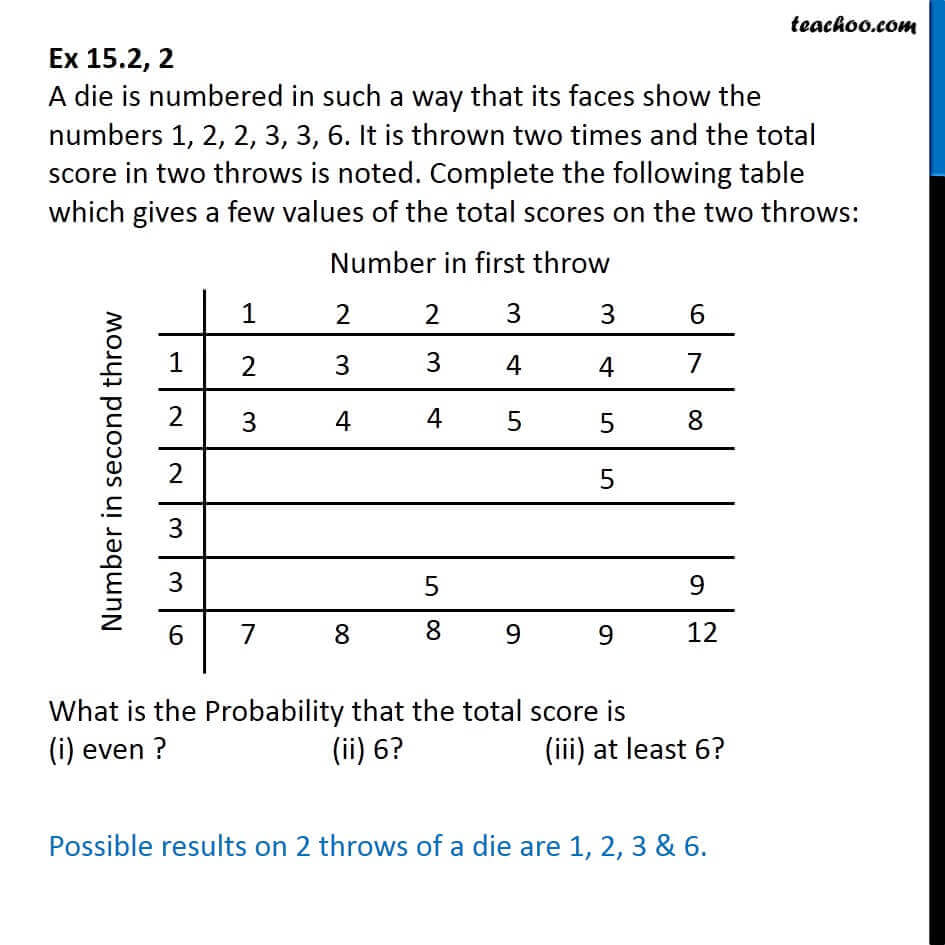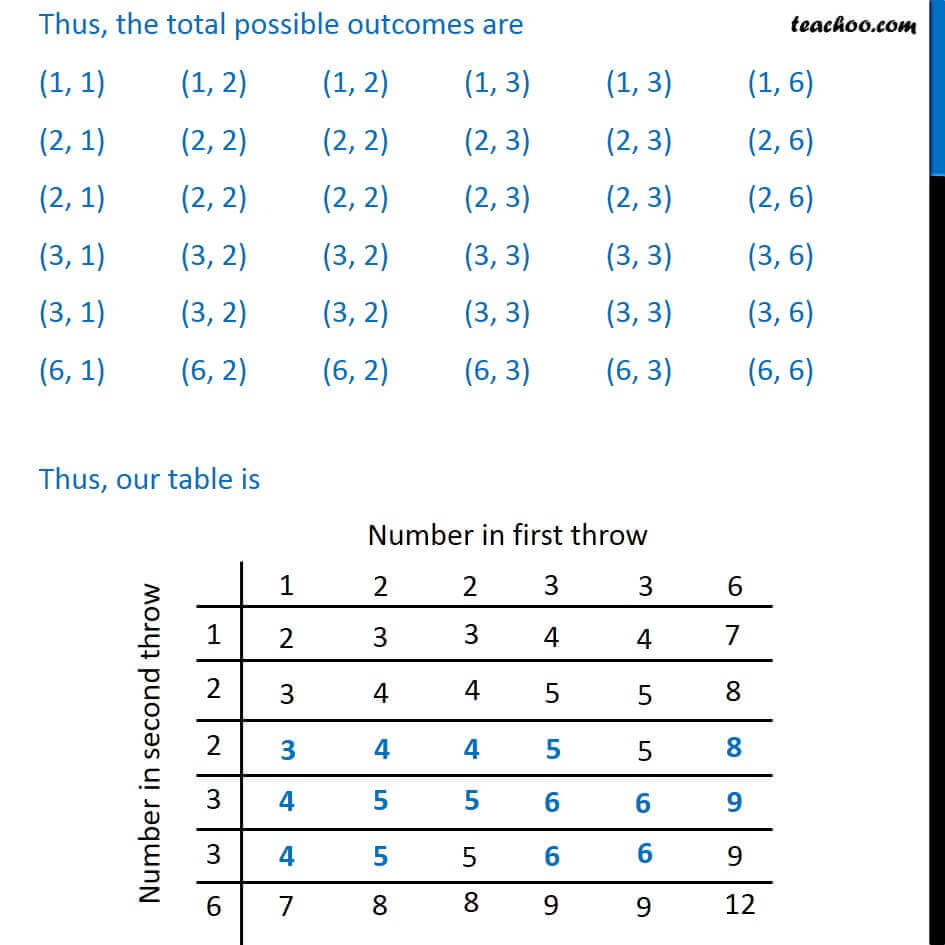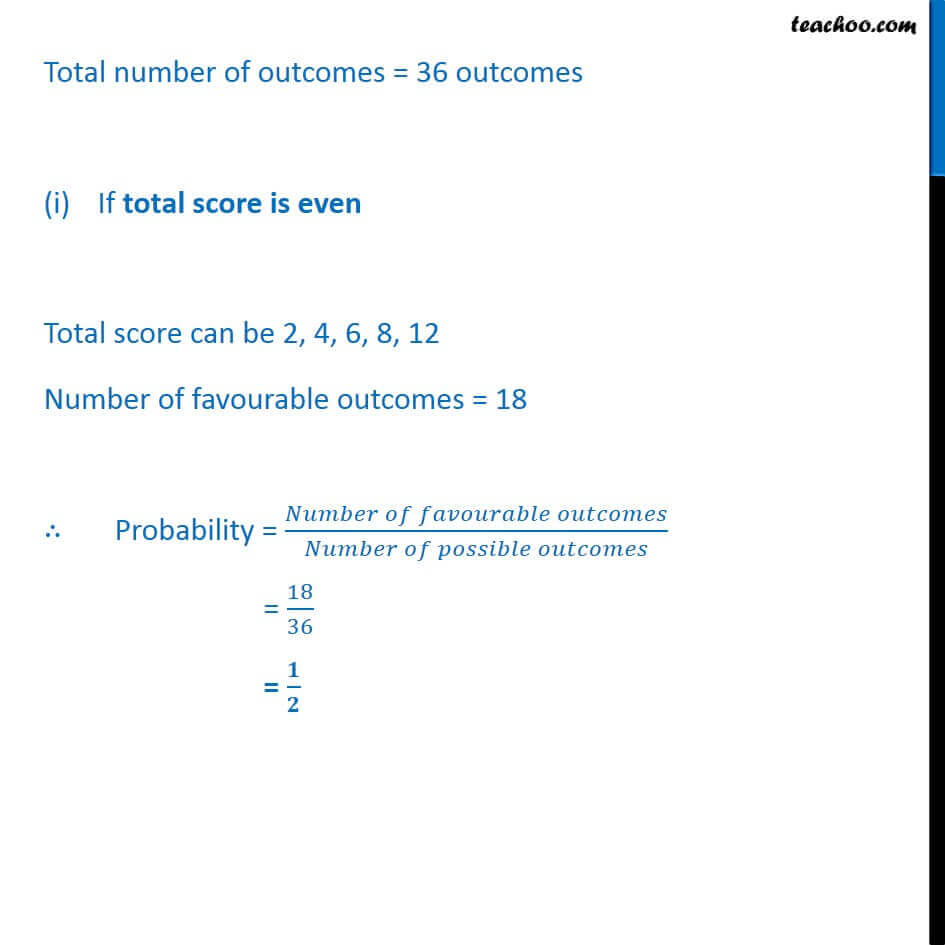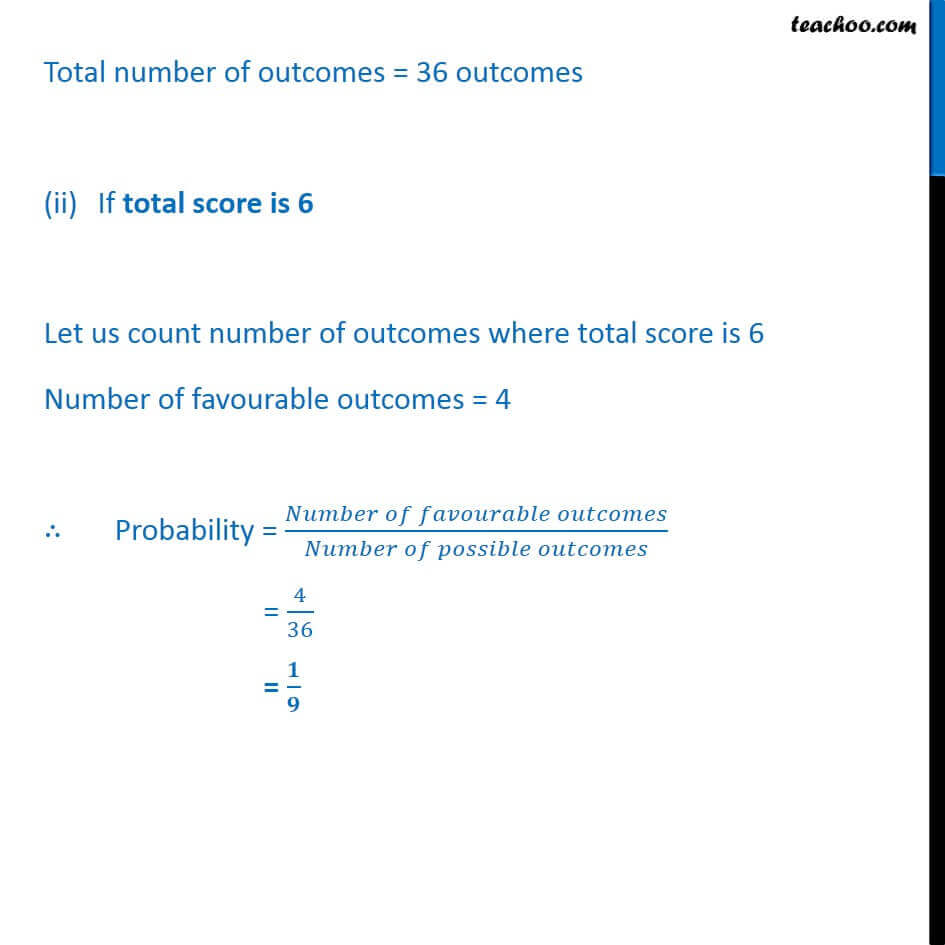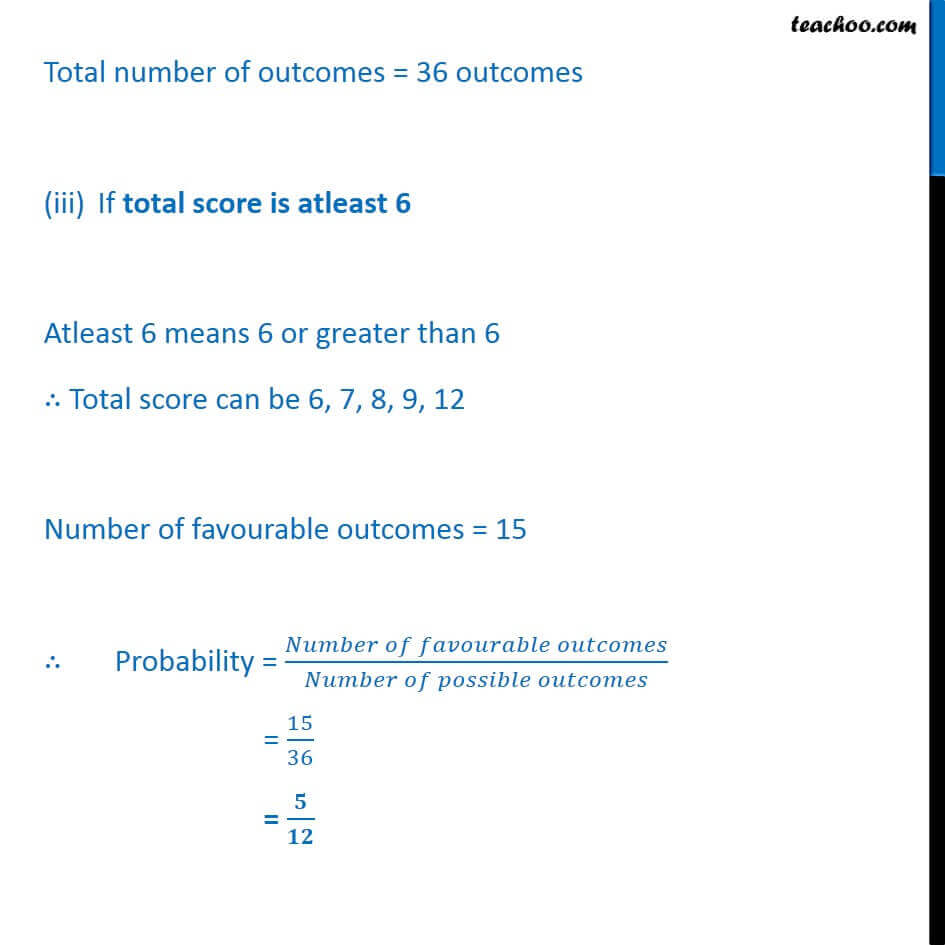Get live Maths 1-on-1 Classs - Class 6 to 12

### Transcript

Ex 15.2, 2 A die is numbered in such a way that its faces show the numbers 1, 2, 2, 3, 3, 6. It is thrown two times and the total score in two throws is noted. Complete the following table which gives a few values of the total scores on the two throws: What is the Probability that the total score is (i) even ? (ii) 6? (iii) at least 6? Possible results on 2 throws of a die are 1, 2, 3 & 6. Thus, the total possible outcomes are (1, 1) (1, 2) (1, 2) (1, 3) (1, 3) (1, 6) (2, 1) (2, 2) (2, 2) (2, 3) (2, 3) (2, 6) (2, 1) (2, 2) (2, 2) (2, 3) (2, 3) (2, 6) (3, 1) (3, 2) (3, 2) (3, 3) (3, 3) (3, 6) (3, 1) (3, 2) (3, 2) (3, 3) (3, 3) (3, 6) (6, 1) (6, 2) (6, 2) (6, 3) (6, 3) (6, 6) Thus, our table is Total number of outcomes = 36 outcomes If total score is even Total score can be 2, 4, 6, 8, 12 Number of favourable outcomes = 18 ∴ Probability = (𝑁𝑢𝑚𝑏𝑒𝑟 𝑜𝑓 𝑓𝑎𝑣𝑜𝑢𝑟𝑎𝑏𝑙𝑒 𝑜𝑢𝑡𝑐𝑜𝑚𝑒𝑠)/(𝑁𝑢𝑚𝑏𝑒𝑟 𝑜𝑓 𝑝𝑜𝑠𝑠𝑖𝑏𝑙𝑒 𝑜𝑢𝑡𝑐𝑜𝑚𝑒𝑠) = 18/36 = 𝟏/𝟐 Total number of outcomes = 36 outcomes If total score is 6 Let us count number of outcomes where total score is 6 Number of favourable outcomes = 4 ∴ Probability = (𝑁𝑢𝑚𝑏𝑒𝑟 𝑜𝑓 𝑓𝑎𝑣𝑜𝑢𝑟𝑎𝑏𝑙𝑒 𝑜𝑢𝑡𝑐𝑜𝑚𝑒𝑠)/(𝑁𝑢𝑚𝑏𝑒𝑟 𝑜𝑓 𝑝𝑜𝑠𝑠𝑖𝑏𝑙𝑒 𝑜𝑢𝑡𝑐𝑜𝑚𝑒𝑠) = 4/36 = 𝟏/𝟗 Total number of outcomes = 36 outcomes If total score is atleast 6 Atleast 6 means 6 or greater than 6 ∴ Total score can be 6, 7, 8, 9, 12 Number of favourable outcomes = 15 ∴ Probability = (𝑁𝑢𝑚𝑏𝑒𝑟 𝑜𝑓 𝑓𝑎𝑣𝑜𝑢𝑟𝑎𝑏𝑙𝑒 𝑜𝑢𝑡𝑐𝑜𝑚𝑒𝑠)/(𝑁𝑢𝑚𝑏𝑒𝑟 𝑜𝑓 𝑝𝑜𝑠𝑠𝑖𝑏𝑙𝑒 𝑜𝑢𝑡𝑐𝑜𝑚𝑒𝑠) = 15/36 = 𝟓/𝟏𝟐